### Home > MC1 > Chapter 9 > Lesson 9.3.1 > Problem9-128

9-128.

Copy and complete each of the Diamond Problems below. The pattern used in the Diamond Problems is shown at right.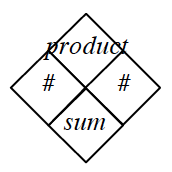1.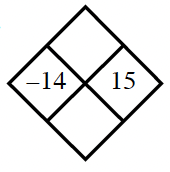Add $x$ and $y$ to get the sum. Multiply $x$ and $y$ to get the product.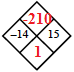1.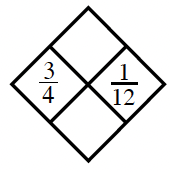Follow the pattern shown above right.

1.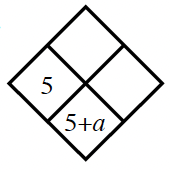$5+ y = 5 + a$
What is $y$?

The product is $5a$.

1.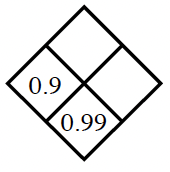$0.99 − 0.90 = 0.09$